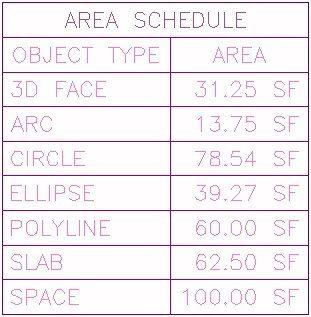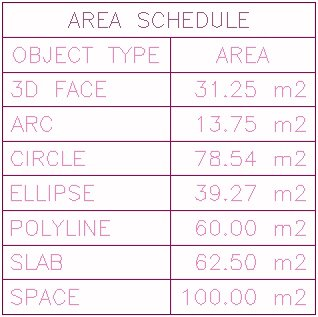## January 25, 2006

### Using System Variable Values in Formula Properties

Have you ever wished you could check the value of an AutoCAD System Variable in a Formula Property? A post in the Autodesk Architectural Desktop 2006 Discussion Group asked if it were possible to set up a conditional Property Data Format that would change the suffix appended to a number, based on the units settings in a drawing. So far as I am aware, that is not possible, but it certainly would be nice to be able to do so.

It occurred to me that if I could read in the value of the MEASUREMENT System Variable, which is set to 0 for imperial units and 1 for metric units, I could then test that value and append the appropriate suffix value in a formula property.

One of the members of the ADT Development team - my apologies, as I do not know to whom to give the credit - had created a formula property for a Door that would read the fire rating property of the Wall to which the Door was anchored. You can get a sample file with that formula, written for ADT 2005, here and read additional discussion on that code here. Starting with that code and hacking my way through the VBA Help, I came up with the following. There are different versions for different ADT releases because the AutoCAD application number changes with each release:

For 2004:
RESULT = app.ActiveDocument.GetVariable("Measurement")

For 2005:
RESULT = app.ActiveDocument.GetVariable("Measurement")

For 2006:
RESULT = app.ActiveDocument.GetVariable("Measurement")

Note that in the actual formula,
RESULT = app.ActiveDocument.GetVariable("Measurement")
is all on one line; it appears on two lines here due to the format of the blog. Substitute the name of any other variable for "Measurement" to get that variable's value. The code above is set up to create a formula property that returns the value of the desired System Variable, and could then be referenced by another formula property. If you only need the value in one formula, you could add the code to the beginning of a formula, replacing RESULT with a variable name which could then be referenced in a conditional statement. For example, in an ADT 2006 drawing, if you had a Property Set Definition that included an automatic property called MyArea that held an unformatted area value, the following would append either " SF" or " m2" to the value in MyArea:

`Set app = GetObject(,"AutoCAD.Application.16.2")unitsType = app.ActiveDocument.GetVariable("Measurement")If unitsType = 0 Then     RESULT = "[MyArea]" & " SF"Else     RESULT = "[MyArea]" & " m2"End If`
I attached a sample file, written for ADT 2004, to the previously mentioned thread in the ADT 2006 Discussion Group that demonstrates how this might be used. In that file, I took a "modular" approach, and have separate formulas to retrieve the MEASUREMENT value, set a suffix string and concatenate the final result. There is a sample Schedule Table showing the results, and if you change the value of MEASUREMENT and then update the Schedule Table, you will see the suffix change between " SF" and " m2".Measurement = 0Measurement = 1

An even cleverer method for the specific issue of area suffixes [or volume suffixes] would be to retrieve the dictionary values of the suffix as set on the Units tab of the Drawing Setup dialog. I am not certain that is possible and I will have to leave chasing that down for another day.